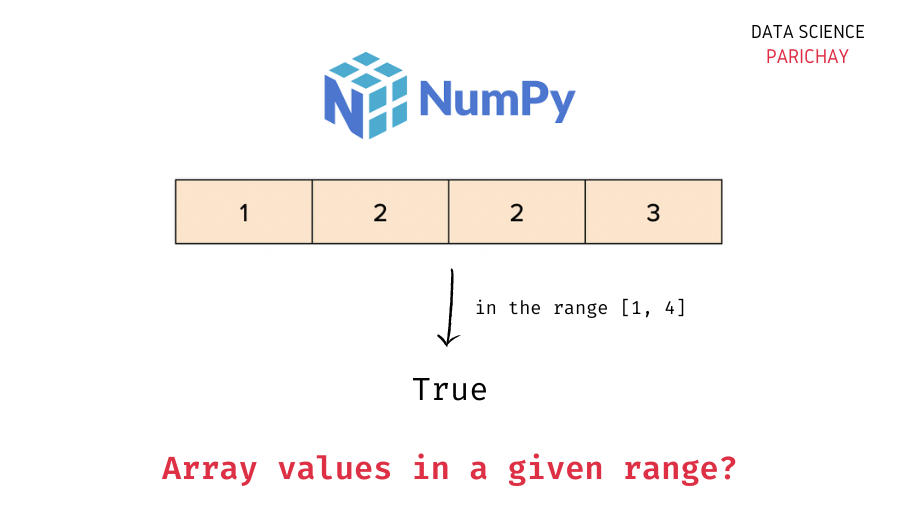# Numpy – Check if Array Values are within a specified Range

In this tutorial, we will look at how to check if all the values in a numpy array are within a specified range with the help of some examples.

## How to check if array elements are within a range?

You can use a combination of comparison operators, the `&` operator and the numpy `all()` function to check if all the values in a numpy array lie within a given range, for example, low to high.

The following is the syntax –

```# check if all values in numpy array in the range [low, high]
((ar >= low) & (ar <= high)).all()```

The idea is to check whether each value in the array is greater than or equal to `low` and less than or equal to `high`. Comparing an array with a scalar value in numpy results in a boolean array, you can combine the results from both the boolean array using the `&` operator to make sure both the conditions are met and then finally check whether all the values in the resulting boolean array are `True` or not using the `all()` function.

If you do not want the range limits to be inclusive, use `>` and `<` instead of `>=` and `<=` respectively.

Let’s now look at some examples of using the above syntax –

## Example – Check array values lie in a range

```import numpy as np

# create a 1d array
ar = np.array([1, 2, 3, 5])

# check if array values lie in the range [0, 7]
print(((ar >= 0) & (ar <= 7)).all())```

Output:

`True`

In the above example, we create an array with some integer values and then check whether all the array values lie in the range [0, 7] or not.

📚 Data Science Programs By Skill Level

Introductory

Intermediate ⭐⭐⭐

🔎 Find Data Science Programs 👨‍💻 111,889 already enrolled

Disclaimer: Data Science Parichay is reader supported. When you purchase a course through a link on this site, we may earn a small commission at no additional cost to you. Earned commissions help support this website and its team of writers.

Alternatively, you can also use the `numpy.logical_and()` method to combine two boolean arrays with an and operation.

```# check if array values lie in the range [0, 7]
print(np.logical_and(ar >= 0, ar <=7).all())```

Output:

`True`

We get the same result as above.

Let’s now take an example, where the condition is violated. For example, in the above array, let’s check if all the values are within the range [0, 4].

```# check if array values lie in the range [0, 4]
# method 1
print(((ar >= 0) & (ar <= 4)).all())
# method 2
print(np.logical_and(ar >= 0, ar <=4).all())```

Output:

```False
False```

We get `False` as the output from both methods which is the correct answer.

You might also be interested in –

•